International
Tables for
Crystallography
Volume F
Crystallography of biological macromolecules
Edited by M. G. Rossmann and E. Arnold

International Tables for Crystallography (2006). Vol. F, ch. 2.1, pp. 52-53   | 1 | 2 |

## Section 2.1.4.1. Diffraction by one electron

J. Drentha*

aLaboratory of Biophysical Chemistry, University of Groningen, Nijenborgh 4, 9747 AG Groningen, The Netherlands
Correspondence e-mail: j.drenth@chem.rug.nl

#### 2.1.4.1. Diffraction by one electron

| top | pdf |

The scattering of an X-ray beam by a crystal results from interaction between the electric component of the beam and the electrons in the crystal. The magnetic component has hardly any effect and can be disregarded.

If a monochromatic polarized beam hits an electron, the electron starts to oscillate in the direction of the electric vector of the incident beam (Fig. 2.1.4.1).This oscillating electron acts as the aerial of a transmitter and radiates X-rays with the same or lower frequency as the incident beam. The frequency change is due to the Compton effect: the photons of the incident beam collide with the electron and lose part of their energy. This is inelastic scattering, and the scattered radiation is incoherent with the incident beam. Compton scattering contributes to the background in a diffraction experiment. In elastic scattering, the scattered radiation has the same wavelength as the incident radiation, and this is the radiation responsible for the interference effects in diffraction. It was shown by Thomson that if the electron is completely free the following hold:

• (1) The phase difference between the incident and the scattered beam is π, because the scattered radiation is proportional to the displacement of the electron, which differs by π in phase with its acceleration imposed by the electric vector.Figure 2.1.4.1 | top | pdf |The electric vector of a monochromatic and polarized X-ray beam is in the plane. It hits an electron, which starts to oscillate in the same direction as the electric vector of the beam. The oscillating electron acts as a source of X-rays. The scattered intensity depends on the angle ϕ between the oscillation direction of the electron and the scattering direction [equation (2.1.4.1)]. Reproduced with permission from Drenth (1999). Copyright (1999) Springer-Verlag.
• (2) The amplitude of the electric component of the scattered wave at a distance r which is large in comparison with the wavelength of the radiation is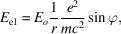whereis the amplitude of the electric vector of the incident beam, e is the electron charge, m is its mass, c is the speed of light and ϕ is the angle between the oscillation direction of the electron and the scattering direction (Fig. 2.1.4.1).Note that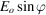is the component ofperpendicular to the scattering direction.

In terms of energy,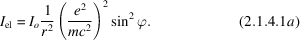The scattered energy per unit solid angle is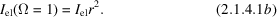It was shown by Klein & Nishina (1929)[see also Heitler (1966)] that the scattering by an electron can be discussed in terms of the classical Thomson scattering if the quantum energy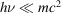. This is not true for very short X-ray wavelengths. For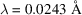,and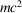are exactly equal, but for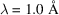,is 0.0243 times. Since wavelengths in macromolecular crystallography are usually in the range 0.8–2.5 Å, the classical approximation is allowed. It should be noted that:

 (1) The intensity scattered by a free electron is independent of the wavelength. (2) Thomson's equation can also be applied to other charged particles, e.g. a proton. Because the mass of a proton is 1800 times the electron mass, scattering by protons and by atomic nuclei can be neglected. (3) Equation (2.1.4.1a)gives the scattering for a polarized beam. For an unpolarized beam,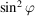is replaced by a suitable polarization factor.

### References

Heitler, W. G. (1966). The quantum theory of radiation, 3rd ed. Oxford University Press.Google Scholar
Klein, O. & Nishina, Y. (1929). Über die Streuung von Strahlung durch freie Elektronen nach der neuen relativistischen Quantendynamik von Dirac. Z. Phys. 52, 853–868.Google Scholar